2016-07-29 00:09:36 yinglang19941010 阅读数 1295
• ###### 自然语言处理——实战分词

1179 人正在学习 去看看 魏俊

HMM处理三种问题，我理解为三种功能
- 给出 O (可观测序列o1 o2 o3… ot），求P(O | hmm)
- 给出 O，求出使P(O, H | hmm)最大的 H （H为与O对应的隐藏层时序序列）
- 给出 O，求出使P(O | hmm) 最大的 hmm（参数调整）

HMM应用于孤立词语音识别流程描述（训练：功能三）
1. 给出a, b, c三个词的音频库（Wa, Wb, Wc）,对音频库的所有样本进行特征提取。（这里以mfcc为例），对每一段音频 w 先分成若干帧[w1,w2, w3…wn]，对每一帧提取mfcc,获得[mfcc1,mfcc2,mffcc3…mfcc_n]。
2. 对这些mfcc进行聚类处理(比如k-means)，获得M个类（类中心），每个类对应一个HMM的可观测层，同时将所有的mfcc进行分类，转化为可观测层的状态，该mfcc归到哪个类就对应哪个可观测状态。（矢量量化）对每一段音频w的mfcc串[mfcc1,mfcc2,mffcc3…mfcc_n]，就会获得它的可观测序列[o1, o2, o3…on]
3. 从而获取了词汇 a ，b ，c 的所有O 序列，记为Oa，Ob，Oc；（Ox表示词汇x的所有样本的可观测层序列向量组成的矩阵）
4. 基于上述的HMM的第三个功能，以Oa，Ob，Oc作为条件，分别获得三个HMM，记为HMMa，HMMb，HMMc；

（识别：功能一）

1. 给出一段孤立词x的音频，对他提取mfcc串,对提取出的mfcc串使用上面聚类的中心进行分类，进而转化为可观测序列记为ox；
2. 基于HMM的第一个功能，将 ox 输入到HMMa，HMMb，HMMc中获得三个概率，记为Pa，Pb，Pc；
3. 比较Pa，Pb，Pc,获取最大的Py(y = a 或 b 或 c ）
4. x = y;

HMM + GMM

bj(X)=m=1MwjmN(X|μjm,Σjm)

X 就直接使用高维特征，比如mfcc。

bj(X)=m=1MwjmN(X|μm,Σm)

2018-05-31 12:30:06 JosephPai 阅读数 3583
• ###### 自然语言处理——实战分词

1179 人正在学习 去看看 魏俊

## 1 从语音识别说起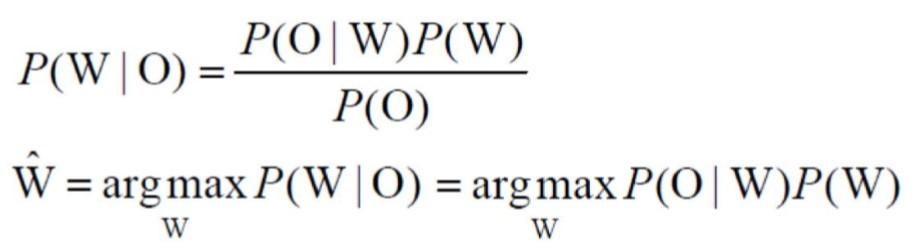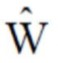： 识别结果
W：任一单词（以孤立词举例说明）
O：输入的语音序列（Observation Sequence）

## 2 计算P(O | W) —— 解码

P(O | W)可以继续分解如下
P(O | W) = P(A, O | W)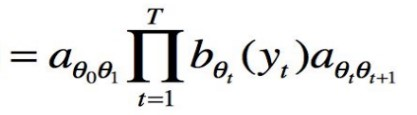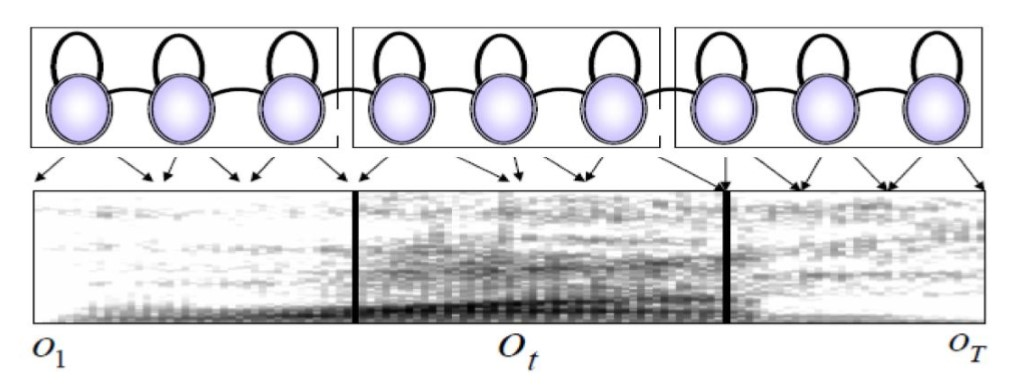## 3 Viterbi算法

Viterbi算法的基础概括成下面三点：

1. 如果概率最大的路径P（或者说是最短路径）经过某点a，那么这条路径上从起始点s到a的这一段子路径一定是s到a之间的最短路径。否则用s到a的最短路径来替换上述路径，便构成了一条比P更短的路径，矛盾。
2. 从S到E的路径必定经过第i时刻的某个状态，假定第i时刻有k个状态，那么如果记录了从S到第i个状态的所有k个节点的最短路径，最终的最短路径必经过其中的一条。这样，在任何时刻，只需要考虑非常有限条最短路径即可。
3. 结合上述两点，假定当我们从状态i进入状态i+1时，从S到状态i上各个节点的最短路径已经找到，并且记录在这些节点上，那么在计算从起点S到前一个状态i所有的k个结点的最短路径，以及从这k个节点到Xi+1，j的距离即可。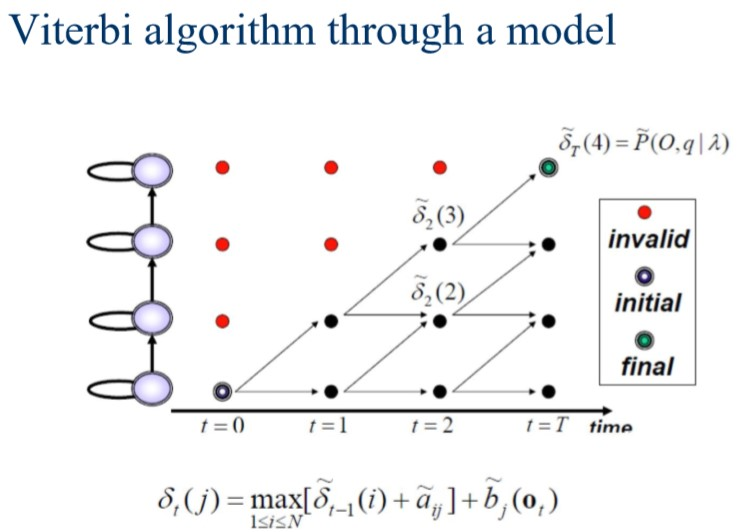Viterbi算法的思想是典型的动态规划思想。动态规划相对穷举已经大大减少了计算量，然而面对巨大的网络，我们意识到还是有很多不必要的计算。有些路径的计算过程中概率已经很小，完全偏离了我们要的最佳路径，继续计算这样的路径显然是没有必要的。这个时候就需要剪枝（pruning），剪枝的Viterbi算法其中一个实现叫做Token Passing，著名的语音识别工具箱HTK的解码部分应用的就是这一概念模型。

## 4 Token Passing Approach

### 4.1 概念模型

初始化（t=0）：
初始state（入口处）的Token的s=0
其他state的Token的s=-inf

复制若干数目Token，并将其传递至所有与该state连接的其他state中，并且对其值做如下操作：
在每个state中，比较所有token，留下分值最高的token，抛弃其他所有token（Viterbi剪枝过程）

比较所有终态（final state）的Token，保留其中分数最高的token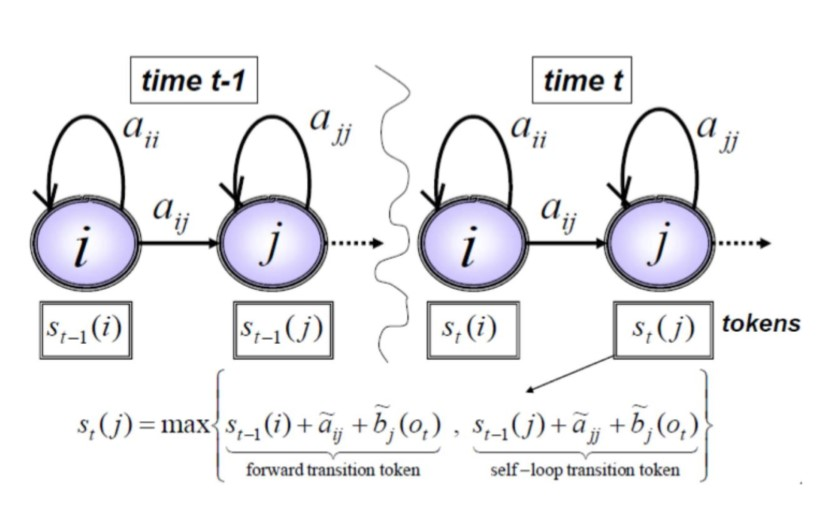### 4.2 孤立词识别（Isolated Word Recognition）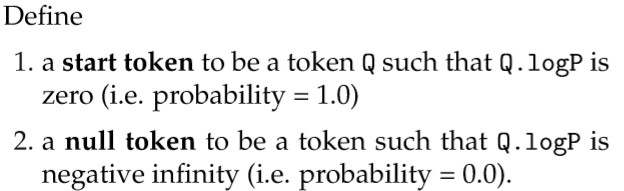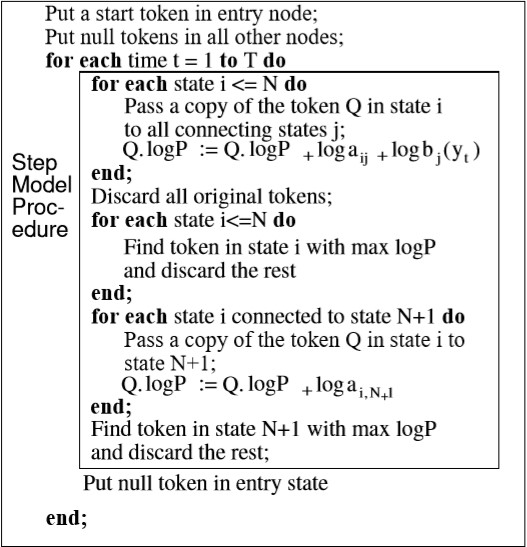### 4.3 连续识别（Connected Word Recognition 或 Continuous Speech Recognition）

① 音频中词与词之间的分界线（boundary）不明确
② 音频序列中总的单词数目不能确定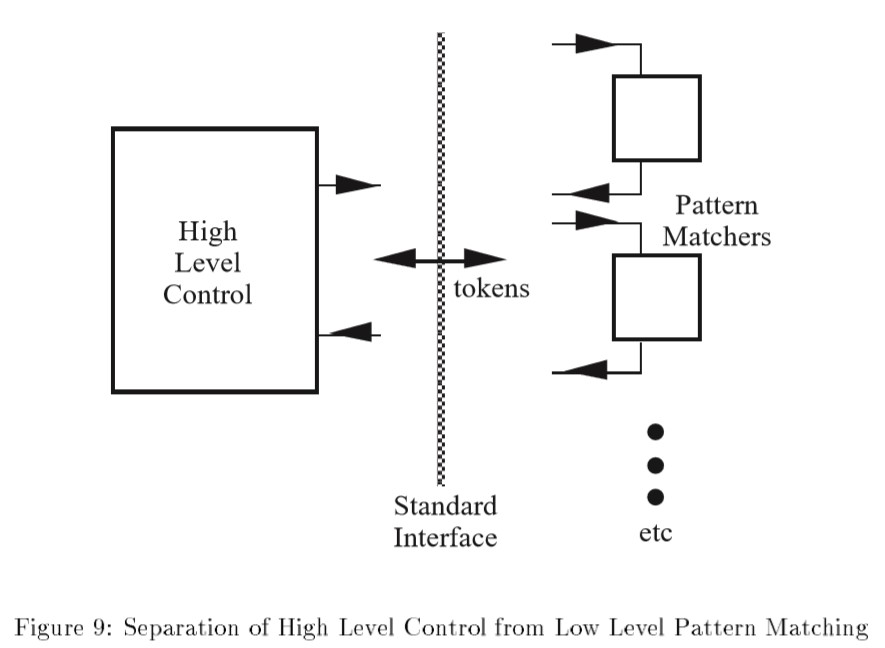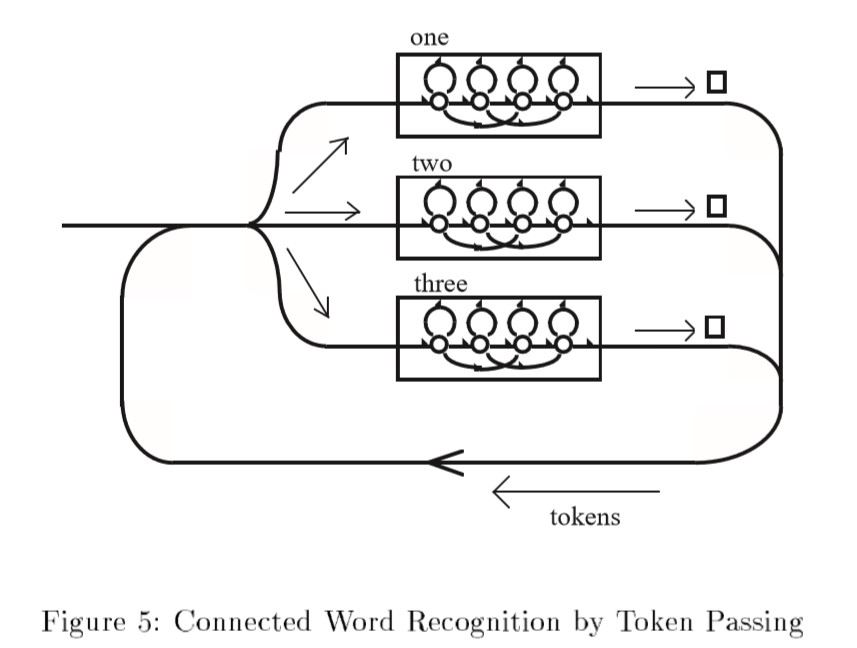struct Token{
double score;
struct WRL *path_id;
}

在时间 t ，对每一个完成单词级识别的token（从小网络传递到大网络） do:
创建一个新的WLR
WLR包含 <token内容（最大概率）， 时间t， 记录路径的path id, 刚刚完成识别的单词model id>
将token中的path id指向刚刚创建的这条新的WLR
end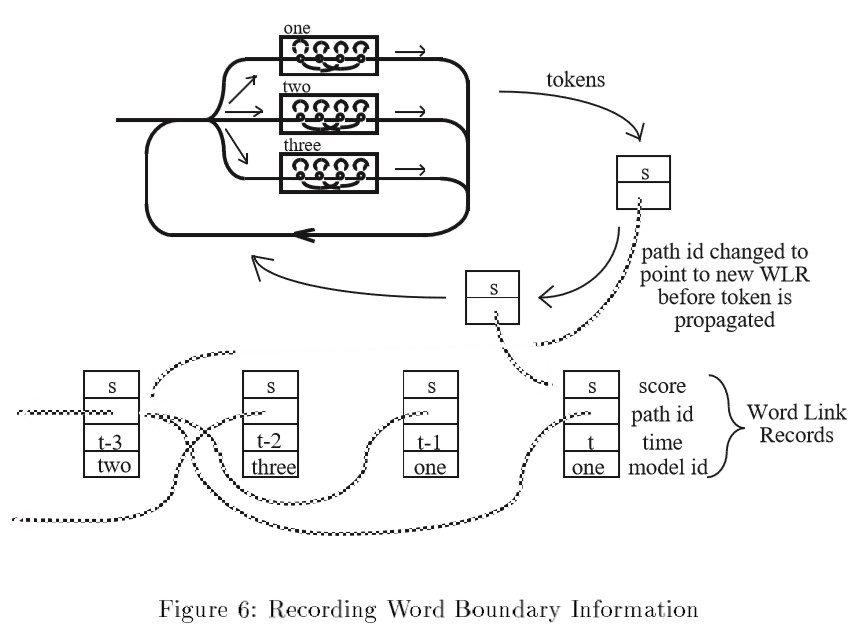### 5 总结

Reference：
 Young S J, Russell N H, Thornton J H S. Token passing: a simple conceptual model for connected speech recognition systems[M]. Cambridge, UK: Cambridge University Engineering Department, 1989.
 Young S, Evermann G, Gales M, et al. The HTK book[J]. Cambridge university engineering department, 2002, 3: 175.
 University of Cambridge Engineering Part IIB & EIST Part II Module 4F11: Speech Processing Lecture 11: Continuous Speech Recognition
 SGN-24006 Analysis of Audio, Speech and Music Signals lec09
 speech.zone

2018-09-30 10:17:11 chinatelecom08 阅读数 4861
• ###### 自然语言处理——实战分词

1179 人正在学习 去看看 魏俊

# 简介

• 本文实现了一个基于HMM-GMM的独立词识别模型，数据集有限，训练数据为独立词，为10类。训练样本100个，测试样本10个，测试集上能够达到90%的识别率。
• 直接下载项目到本地，运行.py文件就可以得到下面的结果，成功执行：
训练集：

['1', '10', '2', '3', '4', '5', '6', '7', '8', '10', '1', '10', '2', '3', '4', '5', '6', '7', '8', '9', '1', '10', '2', '3', '4', '5', '6', '5', '8', '9', '1', '10', '2', '3', '4', '5', '6', '7', '8', '9', '1', '10', '2', '3', '4', '5', '6', '7', '8', '10', '1', '10', '2', '4', '4', '5', '6', '7', '8', '9', '1', '10', '2', '3', '4', '5', '6', '7', '8', '9', '1', '10', '2', '3', '4', '5', '6', '7', '8', '10', '1', '10', '2', '3', '4', '5', '6', '7', '8', '10', '1', '10', '2', '3', '4', '5', '6', '7', '8', '9']

['1', '10', '2', '3', '4', '5', '6', '7', '8', '9', '1', '10', '2', '3', '4', '5', '6', '7', '8', '9', '1', '10', '2', '3', '4', '5', '6', '7', '8', '9', '1', '10', '2', '3', '4', '5', '6', '7', '8', '9', '1', '10', '2', '3', '4', '5', '6', '7', '8', '9', '1', '10', '2', '3', '4', '5', '6', '7', '8', '9', '1', '10', '2', '3', '4', '5', '6', '7', '8', '9', '1', '10', '2', '3', '4', '5', '6', '7', '8', '9', '1', '10', '2', '3', '4', '5', '6', '7', '8', '9', '1', '10', '2', '3', '4', '5', '6', '7', '8', '9']

['1', '10', '2', '3', '4', '5', '6', '7', '8', '3']

['1', '10', '2', '3', '4', '5', '6', '7', '8', '9']
识别率: 0.9


# python建模

## 数据预处理

# -----------------------------------------------------------------------------------------------------
'''
&usage:		准备所需数据
'''
# -----------------------------------------------------------------------------------------------------
# 生成wavdict，key=wavid，value=wavfile
def gen_wavlist(wavpath):
wavdict = {}
labeldict = {}
for (dirpath, dirnames, filenames) in os.walk(wavpath):
for filename in filenames:
if filename.endswith('.wav'):
filepath = os.sep.join([dirpath, filename])
fileid = filename.strip('.wav')
wavdict[fileid] = filepath
# 获取文件的类别
label = fileid.split('_')
labeldict[fileid] = label
return wavdict, labeldict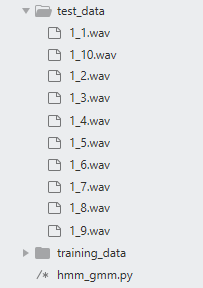wavdict, labeldict = gen_wavlist('test_data')
print(wavdict, labeldict)


wavdict:
{'1_1': 'test_data\\1_1.wav', '1_10': 'test_data\\1_10.wav', '1_2': 'test_data\\1_2.wav', '1_3': 'test_data\\1_3.wav', '1_4': 'test_data\\1_4.wav', '1_5': 'test_data\\1_5.wav', '1_6': 'test_data\\1_6.wav', '1_7': 'test_data\\1_7.wav', '1_8': 'test_data\\1_8.wav', '1_9': 'test_data\\1_9.wav'}
labeldict:
{'1_1': '1', '1_10': '10', '1_2': '2', '1_3': '3', '1_4': '4', '1_5': '5', '1_6': '6', '1_7': '7', '1_8': '8', '1_9': '9'}


## 特征提取

# pip install python_speech_features ，不行的话百度一下
from python_speech_features import mfcc
def compute_mfcc(file):
# 这里我故意fs/2,有些类似减小step，不建议这样做，投机取巧做法
mfcc_feat = mfcc(audio, samplerate=(fs/2), numcep=26)
return mfcc_feat


## 搭建孤立词模型

• 首先，需要初始化10个独立的hmm-gmm模型，分别对应十个独立词，主要是初始化一个hmm-gmm模型的集合 self.models
class Model():
def __init__(self, CATEGORY=None, n_comp=3, n_mix = 3, cov_type='diag', n_iter=1000):
super(Model, self).__init__()
self.CATEGORY = CATEGORY
self.category = len(CATEGORY)
self.n_comp = n_comp
self.n_mix = n_mix
self.cov_type = cov_type
self.n_iter = n_iter
# 关键步骤，初始化models，返回特定参数的模型的列表
self.models = []
for k in range(self.category):
model = hmm.GMMHMM(n_components=self.n_comp, n_mix = self.n_mix,
covariance_type=self.cov_type, n_iter=self.n_iter)
self.models.append(model)


	CATEGORY:	所有标签的列表
n_comp:		每个孤立词中的状态数
n_mix:		每个状态包含的混合高斯数量
cov_type:	协方差矩阵的类型
n_iter:		训练迭代次数

• 然后，用同一种类的数据训练特定的模型。
	# 模型训练
def train(self, wavdict=None, labeldict=None):
for k in range(10):
subdata = []
model = self.models[k]
for x in wavdict:
if labeldict[x] == self.CATEGORY[k]:
mfcc_feat = compute_mfcc(wavdict[x])
model.fit(mfcc_feat)

• 最后，对待测试的数据分别用十个模型打分，选出得分最高的为识别结果。
	# 使用特定的测试集合进行测试
def test(self, wavdict=None, labeldict=None):
result = []
for k in range(self.category):
subre = []
label = []
model = self.models[k]
for x in wavdict:
mfcc_feat = compute_mfcc(wavdict[x])
# 生成每个数据在当前模型下的得分情况
re = model.score(mfcc_feat)
subre.append(re)
label.append(labeldict[x])
# 汇总得分情况
result.append(subre)
# 选取得分最高的种类
result = np.vstack(result).argmax(axis=0)
# 返回种类的类别标签
result = [self.CATEGORY[label] for label in result]
print('识别得到结果：\n',result)
print('原始标签类别：\n',label)
# 检查识别率，为：正确识别的个数/总数
totalnum = len(label)
correctnum = 0
for i in range(totalnum):
if result[i] == label[i]:
correctnum += 1
print('识别率:', correctnum/totalnum)

• 你也可以保存和载入模型
	# 利用external joblib保存生成的hmm模型
def save(self, path="models.pkl"):
joblib.dump(self.models, path)

# 利用external joblib载入保存的hmm模型


## 模型的训练和测试

# 准备训练所需数据
CATEGORY = ['1', '2', '3', '4', '5', '6', '7', '8', '9', '10']
wavdict, labeldict = gen_wavlist('training_data')
testdict, testlabel = gen_wavlist('test_data')
print(testdict, testlabel)
# 进行训练和测试
models = Model(CATEGORY=CATEGORY)
models.train(wavdict=wavdict, labeldict=labeldict)
models.save()
models.test(wavdict=wavdict, labeldict=labeldict)
models.test(wavdict=testdict, labeldict=testlabel)


训练集：

['1', '10', '2', '3', '4', '5', '6', '7', '8', '10', '1', '10', '2', '3', '4', '5', '6', '7', '8', '9', '1', '10', '2', '3', '4', '5', '6', '5', '8', '9', '1', '10', '2', '3', '4', '5', '6', '7', '8', '9', '1', '10', '2', '3', '4', '5', '6', '7', '8', '10', '1', '10', '2', '4', '4', '5', '6', '7', '8', '9', '1', '10', '2', '3', '4', '5', '6', '7', '8', '9', '1', '10', '2', '3', '4', '5', '6', '7', '8', '10', '1', '10', '2', '3', '4', '5', '6', '7', '8', '10', '1', '10', '2', '3', '4', '5', '6', '7', '8', '9']

['1', '10', '2', '3', '4', '5', '6', '7', '8', '9', '1', '10', '2', '3', '4', '5', '6', '7', '8', '9', '1', '10', '2', '3', '4', '5', '6', '7', '8', '9', '1', '10', '2', '3', '4', '5', '6', '7', '8', '9', '1', '10', '2', '3', '4', '5', '6', '7', '8', '9', '1', '10', '2', '3', '4', '5', '6', '7', '8', '9', '1', '10', '2', '3', '4', '5', '6', '7', '8', '9', '1', '10', '2', '3', '4', '5', '6', '7', '8', '9', '1', '10', '2', '3', '4', '5', '6', '7', '8', '9', '1', '10', '2', '3', '4', '5', '6', '7', '8', '9']

['1', '10', '2', '3', '4', '5', '6', '7', '8', '3']

['1', '10', '2', '3', '4', '5', '6', '7', '8', '9']
识别率: 0.9


# hmmlearn安装报错

pip install hmmlearn‑0.2.1‑cp36‑cp36m‑win_amd64.whl


# 一些想法

• 由于基于python的hmm-gmm语音识别模型较少，网上竟然没找到好使的代码，无奈下只能自己查资料写了一个小样。
• 目前该项目只是一个demo，数据也较少，后续希望能够增加一些连续语音识别模型，搞成一个传统模型的语音识别系统。
• 有兴趣的老哥可以查看我的另一个基于深度学习的中文识别系统ch_speech_recognition

2018-01-04 13:47:58 m0_37788308 阅读数 985
• ###### 自然语言处理——实战分词

1179 人正在学习 去看看 魏俊

# 1.语音识别系统的基本结构# 2.涉及算法# 3.GMM高斯混合模型

#### 3.1高斯混合模型的基本概念

高斯混合模型是指具有如下形式的概率分布模型：

p(yθ)=k=1kαkϕ(yθk)

ϕ(yθk)=12πσkexp((yμk)22θ2k)

将二变量的混合高斯分布可以推广到多变量的多元混合高斯分布，其联合概率密度函数可写为：
p(x)=k=1Mcm(2π)(D/2)|m|1/2exp[12(xμm)T1m(xμm)]

=Mm=1CmN(x;μm,μm),(Cm>0)$\qquad\qquad=\sum_{m=1}^{M}{C_{m}N(x;\mu_{m},{\mu}_{m}),\qquad(C_{m}>0)}$

### 3.2用EM算法解决高斯混合分布问题

具体EM算法讲解见另一篇博客：《PLSI主题模型》中EM算法的具体讲解部分：
http://blog.csdn.net/m0_37788308/article/details/78115378
实现EM算法估计高斯混合分布的python代码如下，其中有调用sklearn包进行的估计和直接写的EM算法来估计高斯混合分布。

# -*- coding:utf-8 -*-
#EM算法估计高斯混合分布的参数
import numpy as np
from scipy.stats import multivariate_normal
from sklearn.mixture import GaussianMixture
from mpl_toolkits.mplot3d import Axes3D
import matplotlib as mpl
import matplotlib.pyplot as plt
from sklearn.metrics.pairwise import pairwise_distances_argmin

mpl.rcParams['font.sans-serif'] = [u'SimHei']
mpl.rcParams['axes.unicode_minus'] = False

if __name__ == '__main__':
style = 'myself'
# style = 'sklearn'
np.random.seed(0)
mu1_fact = (0, 0, 0)
cov1_fact = np.diag((1, 2, 3))
data1 = np.random.multivariate_normal(mu1_fact, cov1_fact, 400)
mu2_fact = (2, 2, 1)
cov2_fact = np.array(((1, 1, 3), (1, 2, 1), (0, 0, 1)))
data2 = np.random.multivariate_normal(mu2_fact, cov2_fact, 100)
data = np.vstack((data1, data2))
y = np.array([True] * 400 + [False] * 100)
# print y
if style == 'sklearn':
g = GaussianMixture(n_components=2, covariance_type='full', tol=1e-6, max_iter=1000)
g.fit(data)
print '类别概率:\t', g.weights_
print '均值:\n', g.means_, '\n'
print '方差:\n', g.covariances_, '\n'
mu1, mu2 = g.means_
sigma1, sigma2 = g.covariances_
else:
num_iter = 100
n, d = data.shape
# 随机指定
mu1 = np.random.standard_normal(d)
print mu1
mu2 = np.random.standard_normal(d)
print mu2
mu1 = data.min(axis=0)
mu2 = data.max(axis=0)
sigma1 = np.identity(d)
sigma2 = np.identity(d)
pi = 0.5
# EM
for i in range(num_iter):
# E Step
norm1 = multivariate_normal(mu1, sigma1)
norm2 = multivariate_normal(mu2, sigma2)
tau1 = pi * norm1.pdf(data)
tau2 = (1 - pi) * norm2.pdf(data)
gamma = tau1 / (tau1 + tau2)

# M Step
mu1 = np.dot(gamma, data) / np.sum(gamma)
mu2 = np.dot((1 - gamma), data) / np.sum((1 - gamma))
sigma1 = np.dot(gamma * (data - mu1).T, data - mu1) / np.sum(gamma)
sigma2 = np.dot((1 - gamma) * (data - mu2).T, data - mu2) / np.sum(1 - gamma)
pi = np.sum(gamma) / n
print i, ":\t", mu1, mu2
print '类别概率:\t', pi
print '均值:\t', mu1, mu2
print '方差:\n', sigma1, '\n\n', sigma2, '\n'

# 预测分类
norm1 = multivariate_normal(mu1, sigma1)
norm2 = multivariate_normal(mu2, sigma2)
tau1 = norm1.pdf(data)
tau2 = norm2.pdf(data)

fig = plt.figure(figsize=(13, 7), facecolor='w')
ax.scatter(data[:, 0], data[:, 1], data[:, 2], c='b', s=30, marker='o', depthshade=True)
ax.set_xlabel('X')
ax.set_ylabel('Y')
ax.set_zlabel('Z')
ax.set_title(u'原始数据', fontsize=18)

order = pairwise_distances_argmin([mu1_fact, mu2_fact], [mu1, mu2], metric='euclidean')
print order
if order == 0:
c1 = tau1 > tau2
else:
c1 = tau1 < tau2
c2 = ~c1
acc = np.mean(y == c1)
print u'准确率：%.2f%%' % (100*acc)

ax.scatter(data[c1, 0], data[c1, 1], data[c1, 2], c='r', s=30, marker='o', depthshade=True)
ax.scatter(data[c2, 0], data[c2, 1], data[c2, 2], c='g', s=30, marker='^', depthshade=True)
ax.set_xlabel('X')
ax.set_ylabel('Y')
ax.set_zlabel('Z')
ax.set_title(u'EM算法分类', fontsize=18)
plt.suptitle(u'EM算法的实现', fontsize=21)
plt.tight_layout()
plt.show()

# 4.HMM隐马尔可夫模型

#### 4.1隐马尔可夫的基本概念

隐马尔可夫模型是关于时序的概率模型，描述有一个隐马尔可夫链随机生成不可观测的状态随机序列，再由各个状态生成一个观测而产生观测随机序列过程。隐马尔可的“隐”字主要体现在隐马尔可夫链随机生成的“状态序列”;每一个状态生成一个观察，称为“观察序列”，观察序列我们是可以看到的，但是“状态序列”就是我们常常难理解的地方。
隐马尔可夫由“初始概率分布”、“状态转移概率分布”以及“观察概率分布”这三个分布确定。
Q$Q$所有可能的状态集合，V$V$是所有可能的观测集合。

Q={q1,q2,...,qN}

V={v1,v2,...,vM}

I={i1,i2,...,ir}

O={o1,o2,...,or}

A$A$是状态转移概率矩阵：
A=[aij]NN

B$B$是观测概率矩阵：
A=[bj(k)]NN

bj(k)=p(ot=vk|it=qj),k=1,2,...,M;j=1,2,...,N

π$\pi$是初始状态概率向量：
π=(πi)

πi=p(i1=qi),i=1,2,...,N

λ=(A,B,π)

A,B,π$A,B,\pi$称为隐马尔可夫模型的三要素。

#### 4.2隐马尔可夫的基本假设

(1)齐次马尔科夫性假设，即假设隐藏的马尔科夫链在任意时刻t$t$的状态只依赖与前一时刻的状态，与其他时刻的状态及观测无关，也与时刻t$t$无关。

p(it|it1,ot1,...,i1,o1)=p(it|it1),t=1,2,...,T

(2)观测独立性假设，即假设任意时刻的观测只依赖于该时刻的马尔科夫链的状态，与其他观测状态无关。
p(ot|iT,oT,iT1,oT1,...,it+1,ot+1,it,it1,ot1,...,i1,o1)=p(ot|it)

#### 4.3HMM的三个基本问题

(1)概率计算问题。给定模型π=(A,B,π)$\pi=(A,B,\pi)$和观测序列O=(o1,o2,...,oT)$O=(o_{1},o_{2},...,o_{T})$，计算在模型λ$\lambda$下观测序列O$O$出现的概率p(O|λ)$p(O|\lambda)$
(2)学习问题。已知观测序列O=(o1,o2,...,oT)$O=(o_{1},o_{2},...,o_{T})$，估计模型λ=(A,B,π)$\lambda=(A,B,\pi)$参数，使得在该模型下观测序列概率p(O|λ)$p(O|\lambda)$最大。即用极大似然估计的方法估计参数。
(3)预测问题。也称为解码问题。已知模型λ=(A,B,π)$\lambda=(A,B,\pi)$和观测序列O=(o1,o2,...,oT)$O=(o_{1},o_{2},...,o_{T})$，求对给定观测序列条件概率p(I|O)$p(I|O)$最大的状态序列I=(i1,i2,...,iT)$I=(i_{1},i_{2},...,i_{T})$。即给定观测序列，求最有可能的对应的状态序列。

# 5.GMM-HMM如何应用在语音识别上

解释：这里的语音识别指的是语音的分类，并不是字面意义理解的语音转化为文字。其实这里的语音识别和传统意义上的分类算法一样，通过训练集（train）的训练，对测试集（test）进行分类。只是传统的分类算法输入的是数字数据，而这里的输入的语音。输入的语音信号服从高斯混合分布，也就是隐马尔可夫模型中的第二个问题（学习问题），将语音信号用隐马尔可夫模型中的向前算法（BW算法，也可以理解为EM算法）进行分解，求出隐马尔可夫的三要素（三个参数λ=(A,B,π)$\lambda=(A,B,\pi)$），将参数作为识别语音的特征值，从而进行语音的分类识别。

# 6.问题讲解

(1)在浏览博客中，如果有不理解的地方可以参考视频讲解资料。视频讲解资料链接为：http://v.youku.com/v_show/id_XMzMzMzE1NTY2OA==.html?qq-pf-to=pcqq.c2c
(2)需要《GMM-HMM在语音识别中的应用》的python代码，可以留下邮箱地址。

# 7.参考文献

李航. 统计学习方法[M]. 清华大学出版社:李航, 2012. 171-189

2019-04-07 15:37:37 asrgreek 阅读数 22665
• ###### 自然语言处理——实战分词

1179 人正在学习 去看看 魏俊

##### 我们从下面这段音频说起。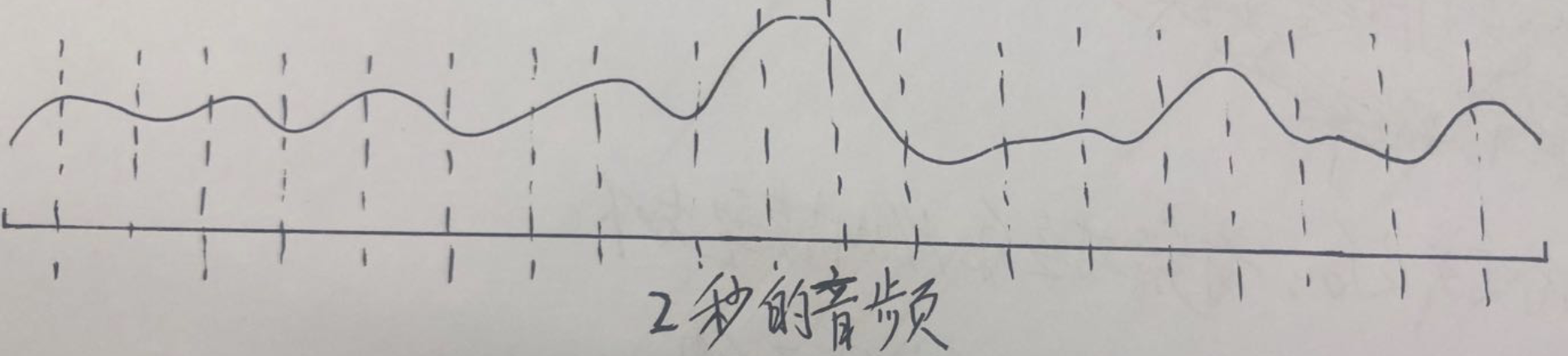###### 一、得到这段音频的标注

1、音素级别的标注。这是训练HMM-GMM模型所需要的标注，也就是说可以直接拿来训练的数据。
2、字节别的标注。实际上我们更多时候拿到是的字级别的标注，因为音素级别的标注工作量很大。但我们可以通过发音词典，将字级别的标注转换成音素级别的标注。这种转换没办法消除多音字的影响，但实际上不会对结果产生太大影响(这一部分可以参考kaldi的compile-train-graphs过程：http://kaldi-asr.org/doc/graph_recipe_train.html)

###### 二、对”n“、”i2“、”h“、”ao3“这4个声韵母分别使用HMM-GMM建模，使用3状态建模，如下图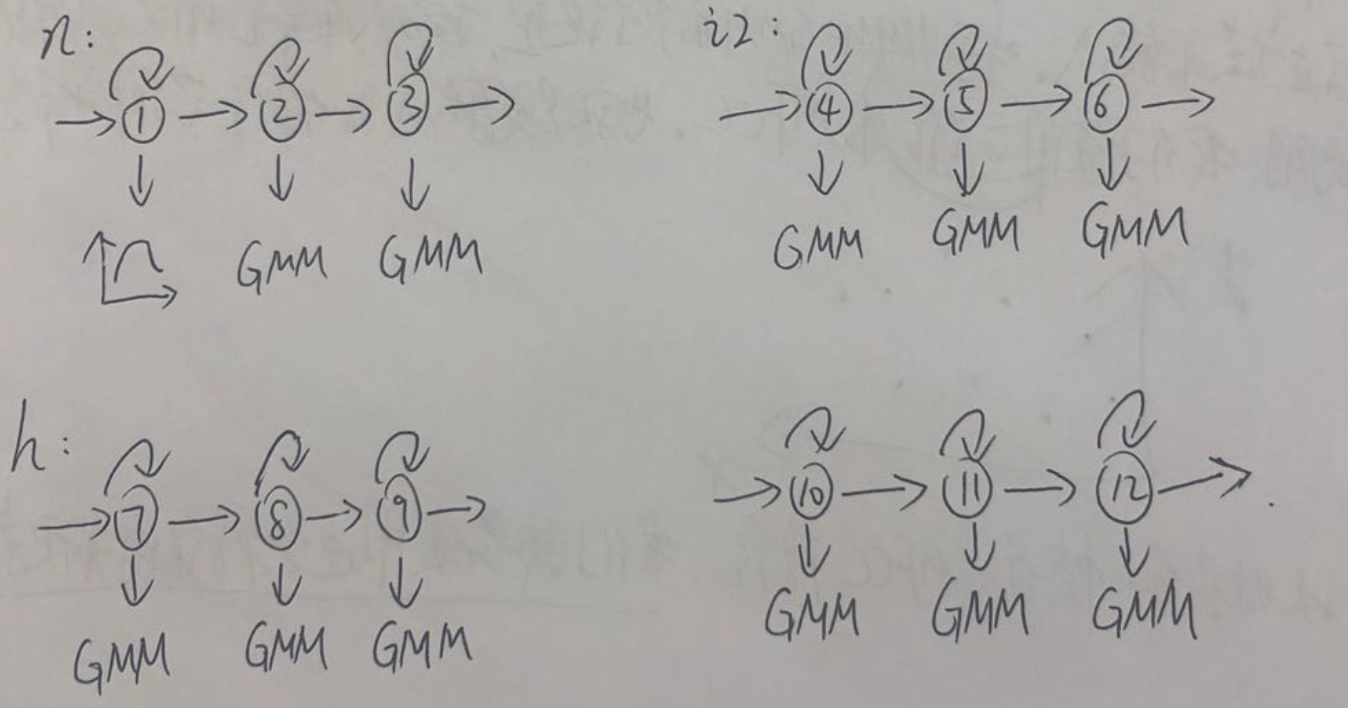###### 三、对”n“、”i2“、”h“、”ao3“对应的HMM-GMM模型进行训练，得到模型参数

• HMM-GMM模型的参数

1、转移概率
2、发射概率：因为我们使用GMM对发射概率建模，所以实际参数就是高斯分布中的均值和方差(这也是初学者容易迷糊的一个地方)。
总结：模型参数就是转移概率、高斯分布的均值、方差

• 模型的输入

输入：每帧信号的MFCC特征(也可以是fbank，plp等)。具体应用中，我们通常取39维的MFCC特征。这里为了方便说明，我们取2维的MFCC特征，也就是说输入的特征空间分布是这样的：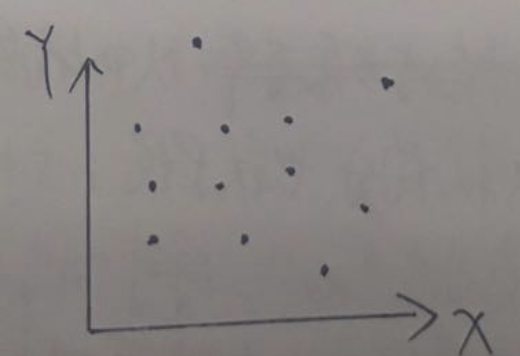上图中，每个黑点对应某一帧信号的MFCC特征。我们需要使用这些特征值来更新GMM的参数，具体怎么更新后续会讲。

• 模型的输出

输出：每一帧属于"n"、“i2”、“h”、"ao3"中的某一个状态(3状态)的概率。

• step1：初始化对齐。以上面的20秒音频为例，因为我们不知道每一帧对应哪个声韵母的某个状态，所以就均分。也就是说1-5帧对应"n"，6-10帧对应"i2"，11-15帧对应"h"，16-20帧对应"ao3"。同时"n"又有三个状态，那么就把1-2帧分给状态①，3-4帧分给状态②，第5帧分给状态③。“i2”、“h”、"ao3"亦如此。这里每个状态只是用单高斯描述，如果是混合高斯，还需要进一步用k-means算法来对每个高斯分量初始化。
初始化完成后，该段音频对应的HMM模型如下：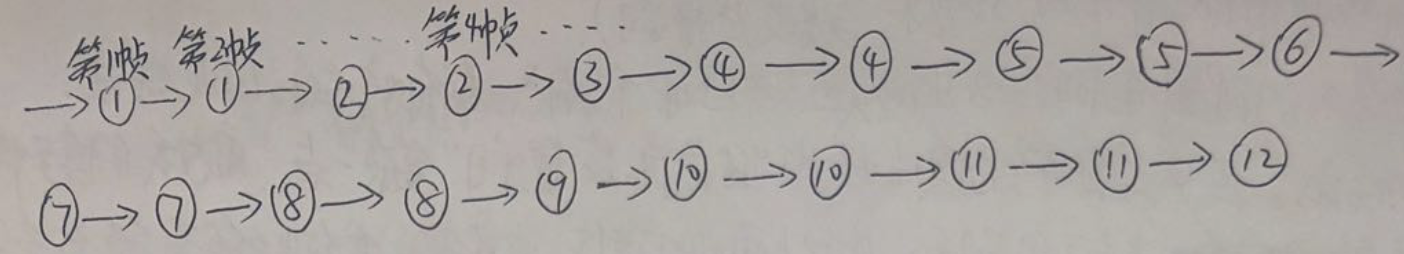• step2：更新模型参数。
1、转移概率：通过上图，我们可以得到①->①的转移次数，①->②的转移次数等等。然后除以总的转移次数，就可以得到每种转移的概率，这是一个统计的过程，无他。
2、发射概率：即均值和方差。以状态①的均值和方差为例，由上图我们可以知道第1帧和第2帧对应状态①。假设第1帧的MFCC特征是(4,3)，第2帧的MFCC特征的MFCC特征是(4,7)。那么状态①的均值就是(4,5)，方差是(0,8)

• step3：重新对齐。根据step2得到的参数，重新对音频进行状态级别的对齐。这一步区别于step1的初始化，step1的初始化我们是采用粗暴的均匀对齐，而这一步的对齐是根据step2的参数进行对齐的。这里的对齐方法有两种：a、硬对齐：采用维特比算法；b、软对齐：采用前后向算法。
经过重新对齐后，可能变成这样(第2帧已经不对应状态①了)：• step4：重复step2和step3多次，直到收敛。